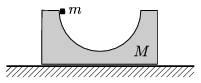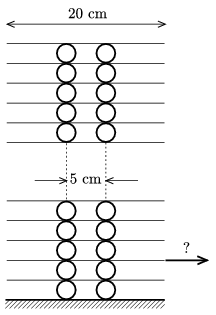Mathematical and Physical Journal
for High Schools
Issued by the MATFUND Foundation
 Already signed up? New to KöMaL?

# KöMaL Problems in Physics, December 2011

Show/hide problems of signs:## Problems with sign 'M'

Deadline expired on January 10, 2012.

M. 319. Make sodium cloride solutions in water of different mass concentration as 5%, 10%, and 15%. Cool down the solutions (for example in the freezer) and investigate at what temperature will the solutions begin to freeze.

(6 pont)

statistics## Problems with sign 'P'

Deadline expired on February 10, 2012.

P. 4391. A dog is at a distance of 40 m from its owner. If they move towards each other then they meet after 10 seconds. If they move in the same direction, then the dog reaches the owner in 20 seconds. Find the speed of the dog and the owner. Suppose that their motion is uniform straight line motion.

(3 pont)

solution (in Hungarian), statistics

P. 4392. A long vertical copper tube hangs on a spring balance. A small bar magnet is placed into the rod and it falls through the tube, such that it does not touch the wall of the tube. Will the reading on the spring balance change?

(4 pont)

solution (in Hungarian), statistics

P. 4393. At the Olympic games in 1984 the British Daley Thompson won the decathlon gold medal. (See here.) (He also won the gold medal in 1980.) His results were the following: 10.45 seconds in 100 metres and 5 m in pole vault. In which case did he have more mechanical energy?

(4 pont)

solution (in Hungarian), statistics

P. 4394. A semi-cylinder is cut out of a block and the block is fixed on the horizontal surface. A small body of mass m is released at the top of the cylinder shaped hole. (Friction is negligible.)a) What is the force that the small body exerts on the block at the lowest point of its path?

b) If the block is able to move on the horizontal surface without friction, then the force exerted on the block by the small body at the lowest point is. By what fraction is the mass of the block greater than the mass of the small body?

(5 pont)

solution (in Hungarian), statistics

P. 4395. In a container there is a sample of Hydrogen gas, its gauge pressure is 400 Pa. If a small valve (its diameter is some millimetres) at the top of the container is opened, then the initial speed of the leaking Hydrogen is 100 m/s. What was the temperature of the Hydrogen? (The ambient air pressure is 1 bar.)

(4 pont)

solution (in Hungarian), statistics

P. 4396. Given n voltaic cells of electromotive force U0 and of internal resistance Rb each. Find the greatest power available from the cells if they are a) connected in series; b) connected in parallel.

(4 pont)

solution (in Hungarian), statistics

P. 4397. News in the dog days: In summer the transmission lines get longer, thus the electrical energy that can be transmitted through them decreases.'' Investigate the truth content of this news.

(4 pont)

solution (in Hungarian), statistics

P. 4398. An inductor with a self-inductance of 36 mH and an unknown one to be wound opposite are connected in series. The coefficient of coupling between the coils is given,. Find the self-inductance of the unknown coil to have a mmaximum current in the circuit.

(5 pont)

solution (in Hungarian), statistics

P. 4399. An object of size 2 cm is placed 40 cm from a glass lens, close to the principal axis of the lens, and the lens forms an image of size 2 cm. What is the size of the image and what type of image is formed if the lens and the object, in the same arrangement, is immerged into water? The refractive index of the lens is 1.5, and that of the water is 1.33.

(4 pont)

solution (in Hungarian), statistics

P. 4400. William Ramsay a British chemist observed that the one of the optical spectral lines of the gas which was liberated from the mineral cleveite, containing uranium, is the same as theline in the spectrum of the Sun. Which gas did Ramsay find? How did this gas originate from the mineral?

(3 pont)

solution (in Hungarian), statistics

P. 4401. A tall tower is built from alike tubes, which have thin walls, and from rectangular sheets, which have negligible mass with respect to that of the tubes, as shown in the figure. What will happen if one of the sheets at the bottom is dynamically pulled out along the horizontal, perpendicularly to the tubes a) by 2 cm; b) by 6 cm? (Static friction is big enough, such that the tubes does not slip.)(6 pont)

solution (in Hungarian), statistics